# ICSE Class 9 Physics Sample Question Paper 3 with Answers

## ICSE Class 9 Physics Sample Question Paper 3 with Answers

Section-1
(Attempt all questions from this section)

Question 1.
(a) A sensitive instrument can measure lengths up to an accuracy of 1 p. Upto how many decimal places would we express the result of a length measurement, done with this instrument, in cm ?
(b) Define the astronomical unit (AU). Express it in metre.
(c) Fill in the blanks :
(i) The time interval between two successive passages of the earth, through the vernal equinox, is known as the …………….
(ii) The atomic unit of mass equals …………….  of the mass of an atom of the carbonisotope $${ }_{6}{ }^{12} \mathrm{C}.$$
(d) Out of the following, which years would be leap years :
1952, 1966, 1978, 1992, 2000 ?
(e) The length of a given second’s simple pendulum is increased to 4 times its original value. What would be its new time period ?
(a) We have, 1 p = 10-6 m = 10-6 x 102 cm = 10-4 cm
The least count of the instrument is therefore, 0.0001 cm. The results of measurements, done with this instrument, would be expressed up to four decimal places, in cm.(b) One astronomical unit (AU) is equal to the mean distance between the earth and the sun.
1 AU = 1.496 x 1011 m

(c) (i) Tropical Year
(ii) $$\frac{1}{12} \mathrm{th}$$

(d) 1952, 1992 and 2000.

(e)The new time period will thus be 4 second.

Question 2.
(a) What do we understand by free fall ?
(b) Fill in the blanks :
(i) The acceleration due to gravity is always directed …………………. downwards.
(ii) The name given to the change in velocity of a particle in one second is ……………….
(c) A cyclist covers a distance of 1.5 km with a uniform speed of 6 m/s. How much time does he take ?
(d) What is the initial velocity and acceleration of the particle whose velocity-time graph is as shown in the figure below ?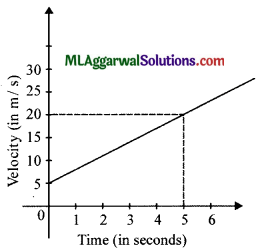(e) A particle is thrown vertically upwards with an initial velocity u. Find the time taken by it to reach the highest point of its path.
(a) When an object falls freely, under the gravitational pull of the earth, without any resistance to its motion, it is said to have a free fall. All freely falling objects (irrespective of their weight) fall towards the earth with the same acceleration.

(b) (i) Vertically
(ii) Acceleration.(c) We have,
Distance = Speed x Time
$$\text { Time }=\frac{\text { Distance }}{\text { Speed }}=\frac{1.5 \times 1000}{6}$$
= 250 s = 4 minute 10 s.

(d) The initial velocity of the particle is clearly 5 ms-1
The acceleration equals the slope of the graph.
Now $$\text { Slope }=\frac{20-5}{5-0}=\frac{15}{5}=3$$
∴ The acceleration of the particle is 3 ms-2.

(e) At the highest point, the velocity of the particle becomes zero.
Using v = u + at
We have, in this case, v = 0 , u = ? r a = – g
0 = u – gt or t = $$\frac{u}{g}$$

Question 3.
(a) When the branches of an apple tree are shaken strongly, the apples fall down. Why ?
(b) Why do skiers use flat and long ‘skis’ ?
(c) Do gases also exert an upthrust on objects kept in them ?
(d) An object is put at a depth of 10 m inside a liquid having the same density as that of the object. What is likely to happen to the object ?
(e) A given test tube is loaded with lead shots and is found to weigh 25 gf in air. It floats upto a certain mark ‘x’ in water. When it is next put in a brine solution, it floats upto the same mark V only when 5 g more of lead shots are added to the test tube. What is the relative density of the brine solution ?
(a) This is because of inertia of rest. When the branches are shaken strongly, the apples are left behind because of their inertia of rest and therefore, fall down.

(b) The use of flat and long ‘skis’ increases the area over which the weight of the skier acts. This decreases their pressure on the snow and helps them to slide smoothly.

(c) Yes, gases, like liquids, also exert an upthrust on objects kept in them. However, because the density of gases is very low, the upthrust, due to them, is usually very small. It is therefore, often neglected.(d) The object will just stay where it has been put. It will neither rise nor sink. This is because the densities of the body and liquid are equal and so the upthrust is equal to the weight of the body. Hence, the net force on it (weight – upthrust) is zero.

(e) The floating tube displaces equal volumes of water and brine solution when its weights are 25 gf and
(25 + 5) 30 gf respectively.
∴ R.D. of brine solution $$=\frac{\text { Weight of a given volume of brine solution }}{\text { Weight of an equal volume of water }}$$
$$=\frac{30}{25}=1.2 \mathrm{gf}$$

Question 4.
(a) What do you mean by anomalous expansion of water ?
(b) Name two substances which expand on heating.
(c) State and draw diagrams to show the difference between regular and irregular reflection.
(d) The frequency of a sound wave of wavelength 8 cm equals 4000 Hz. Find the speed of propagation of this sound wave.
(e) Draw a diagram showing the temperature of various layers of water in an ice covered pond.
(a) The expansion of water when it is cooled from 4°C to 0°C is known as anomalous expansion of water.
(b) Two substances which expand on heating are :

• Brass
•  Iron

(c)(d) We know, ν =fλ
Speed = Frequency x Wavelength
= 4000 Hz x $$\frac{8}{100}$$
= 320 ms-1(e)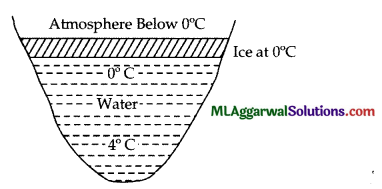Section – II
(Attempt any four questions from this section)

Question 5.
(a) Up to how many decimal places should we express the result (in cm) of a length measured with
(i) a meter scale
(ii) vernier calliper and
(iii) screw gauge ?
(b) An instrument has 20 division on vernier scale which coincide with 19th division on the main scale. If one centimetre on main scale is divided into 20 parts, find its least count.
(c) A screw gauge has a negative error of 5 divisions. While measuring the diameter of a wire, the reading on the main scale is 3 divisions, with the 9th circular scale division coinciding with the base line. If the main scale has 10 divisions to a centimetre and the circular scale has 100 divisions, calculate :
(i) pitch
(ii) least count
(iii) observed diameter, and
(iv) corrected diameter.
(a) (i) Upto the first decimal place only.
(ii) Upto two decimal places.
(iii) Upto three decimal places.(b) Value of one division on main scale (s) = $$\frac{1}{20}cm$$ = 0.05 cm
Total number of divisions on vernier scale (n) – 20
∴ Least count of vernier callipers $$=\frac{\text { Value of one smallest division on main scale }(s)}{\text { Total number of division on vernier scale }(n)}$$
$$=\frac{0.05}{20} \mathrm{~cm}=0.0025 \mathrm{~cm}$$

(c)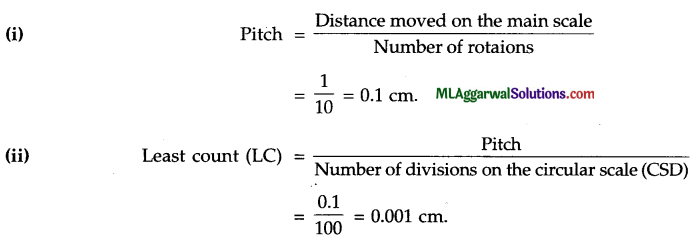(iii) Observed diameter of wire = Main scale reading + LC x CSD coinciding
= 0.3 + 0.001 x 9 = 0.309 cm

(iv) Negative error = 5 divisions Negative correction = LC x 5 divisions
= (0.001 x 5) = 0.005
Corrected diameter = 0.309 + 0.005 = 0.314 cm.

Question 6.
(a) A particle is thrown vertically upwards with an initial velocity. Find the maximum height 7i’ to which the particle will rise.
(b) Gravitational force on the surface of the moon is only 1/6th as strong as gravitational force on earth. What is the weight in newton of a 20 kg body on the moon and on the earth ? (Take g = 9.8 ms-2)
(c) The displacement-time graph of a body is as shown in the figure. Find its average speed over each of the intervals OA, AB, BC, CD.(a) When the particle reaches its maximum height, its velocity becomes zero. Also, the upward moving particle is acted upon by a downward acceleration, the acceleration due to gravity. It therefore, experiences a retardation and its velocity finally becomes zero at the highest point.
Using   v2 – u2 = 2as
where v = 0, u = u, a = – g, and s = h
i.e., the particle has a retardation and s = h.
Thus,  02 – u2 = 2(- g)h
$$h=\frac{u^{2}}{2 g}$$
Therefore, the maximum height attained by the particle is $$\frac{u^{2}}{2 g}$$Question 7.
(a) (i) Write down the formula for pressure at a given point in a liquid and explain the various symbols used.
(ii) A solid cube of dimensions 50 cm x 50 cm x 50 cm and weighing 25 N is placed on a table. Calculate the pressure exerted on the table.
(b) The relative density of a body is 0.52 and its volume is 2 m3. What is the mass of the body ?
(c) A flat bottomed test tube was filled with some lead shots and was found to weigh 20 g in air. This tube was observed to float with (i) 10 cm of its length immersed in water and (ii) 12 cm of its length immersed in kerosene. Find the :
(i) Mass of water displaced by the tube when it is floating in water.
(ii) Area of cross-section of the tube, and
(iii) D. of kerosene.
(a) (i) The formula for pressure is : P = hρg, where T’ denotes pressure, ‘h’ denotes the (vertical) height of the liquid column, ‘ρ’ is the density of the liquid, and ‘g’ is acceleration due to gravity at a given place.

(ii) The weight of the cube = 25 N; area = (50 x 50) cm2 = 2500 x 10-4 m2 = 0.25 m2
$$\text { Pressure }=\frac{\text { Force }}{\text { Area }}=\frac{25 \mathrm{~N}}{0.25 \mathrm{~m}^{2}}$$
= 100 Nm-2 = 100 pascal

(b) We Know that $$\text { R.D. }=\frac{\text { Density of a body }}{\text { Density of water }}$$
∴ Density of body = 0.52 x 1000 kg m 3
= 520 kg m-3
Volume of body = 2 m3

(c)  (i) When the tube is floating in water, displaced by it, must equal the mass of the tube itself. Hence, mass of water, displaced by the tube, when it is floating in water = 20 g.(ii) Let A (in m2) be the area of cross-section of the tube. As it dips 10 cm in water (of density 1000 kg m-3), the volume of water displaced by it is :
$$\mathrm{V}=\mathrm{A} \times\left(\frac{10}{100} \mathrm{~m}\right)=0.1 \mathrm{~A} \mathrm{~m}^{3}$$

∴ Mass of water displaced by it is
= V x Density
= 0.1 A m3 x 1000 kg/m3
= 100 A kg
This equals 20 g (20 x 10-3 kg)
Hence,  100 A = 20 x 10-3
A = 2 x 10-4 m2 = 2 cm2

(iii) Since the tube dips 12 cm in kerosene, the volume of kerosene displaced by it is
V = A x (12 x 10-2) m3 = 2 x 12 x 10-6 m3
If ρ is the density of kerosene, we must have mass of kerosene displaced
= 2 x 12 x 10 x ρ kg
This must again equal 20 g (20 x 10-3 kg)
Hence,  24 x 10-6 x ρ = 20 x 10-3Question 8.
(a) Draw a neat diagram to show the formation of image of an extended object placed in front of a plane mirror.
(b) What are the characteristics of the image formed by a plane mirror ? How is the position of image related to the position of object ?
(c) What is meant by lateral inversion of an image in a plane mirror ? Which letters of the English alphabet do not show lateral inversion ?
(a) Image of an extended object formed by a plane mirror :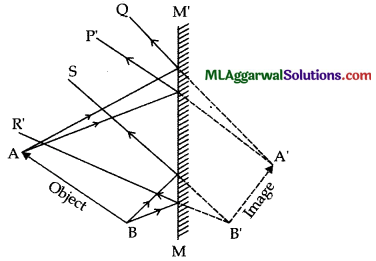(b) The image formed by a plane mirror is (1) upright (or erect), (2) virtual, (3) of the same size as the object, and (4) laterally inverted. The image is situated at the same perpendicular distance behind the mirror as the object is in front of it.

(c) The interchange of the left and right sides in the image of an object formed by a plane mirror is called lateral inversion. The letters of the English alphabet not showing lateral inversion are :
A, H, I, M, O, T, U, V, W, X and Y.

Question 9.
(a) (i) In which of the three media, air, water or iron, does sound travel the fastest at a particular temperature (b) (ii) A person has a hearing range from 20 Hz to 20 kHz. What are the typical wavelengths of sound waves in air corresponding to these two frequencies? Take the speed of sound in air as 344 ms-1.

(b) (i) What is the audible range of the average human ear?
(ii) How is ultrasound used for cleaning?

(c) A wave pulse of frequency 300 Hz, on a string moves a distance of 6m is 0.05 s. Calculate
(i) Velocity of the pulse, and
(ii) Wavelength of wave on string.
(a) (i) Sound travels fastest in iron as compared to water and air.
(ii) Wavelength of sound of frequency 20 Hz is $$\lambda_{1}=\frac{v}{f_{1}}=\frac{344}{20}=17.2 \mathrm{~m}$$
Wavelength of sound of frequency 20 kHz is $$\lambda_{2}=\frac{v}{f_{2}}=\frac{344}{20000}=0.0172 \mathrm{~m}$$

(b) (i) An average human ear can sound waves between frequencies 20 Hz to 20,000 Hz.
(ii) The object to be cleaned is put in a tank fitted with ultrasonic vibration. The tank is filled with water containing detergent. As the ultrasonic vibration is switched on, the detergent rub against the object at a very high frequency and hence cleans it.(c) Given: d = 6 m, t = 0.05 s,f= 300 Hz.
(i) The velocity of pulse,Question 10.
(a) (i) What is an electromagnet ?
(ii) State any two ways of increasing the magnetic field of an electromagnet.
(iii) Name three constituents of an atom and state the nature of charge on each of them.
(iv) In the electric circuit shown in the figure, name the different parts A, B, C, D, E and F. Show in the diagram the direction of flow of current.
(i) An electromagnet is a temporary strong magnet made from a piece of soft iron. when current flows in the coil wound around it.

(ii) The magnetic field of an electromagnet can be increased by :

• Increasing the number of turns of winding in the solenoid, and
• By increasing the current through the solenoid.

(b) The three constituents are

• Neutron
• Proton
• Electron.

Neutron is neutral, proton has positive charge and electron has a negative charge.(c) A-ammeter, B-cell, C-key, D-resistor, E-voltmeter and F-rheostat.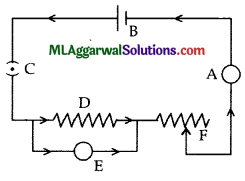Categories ISC